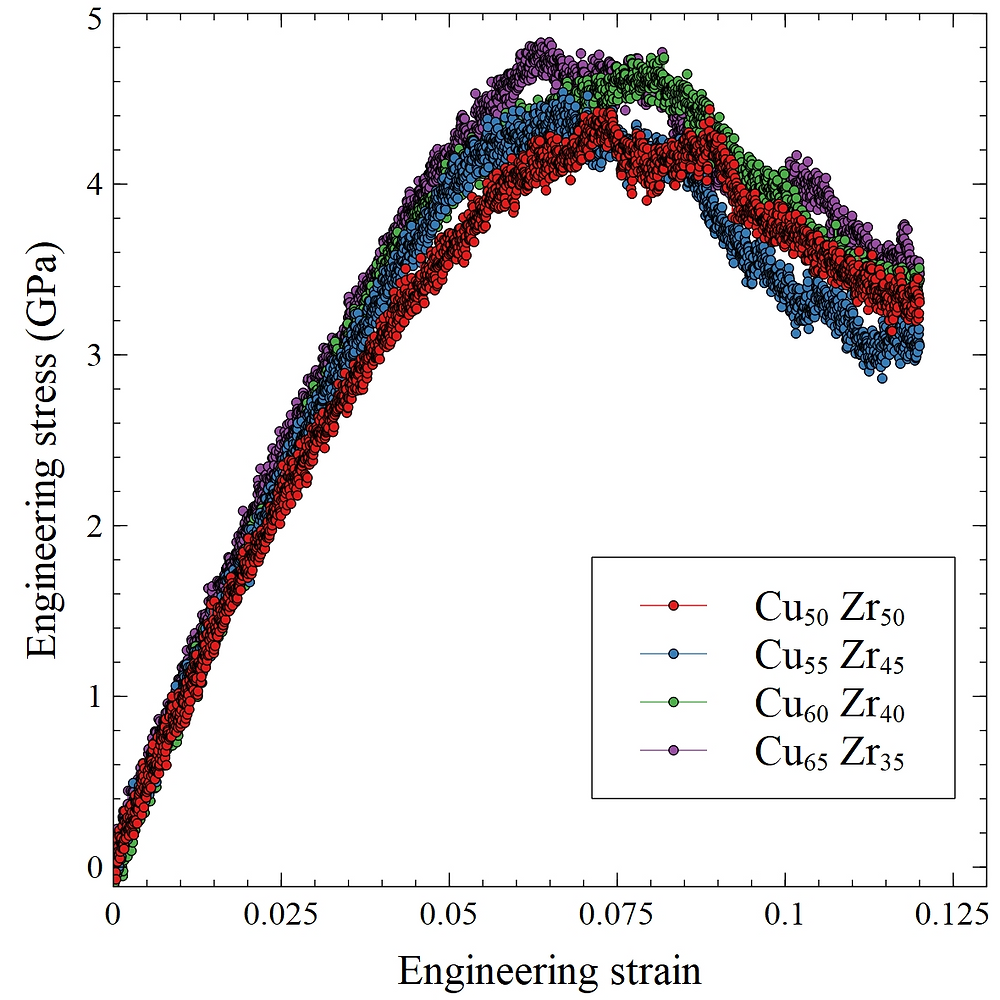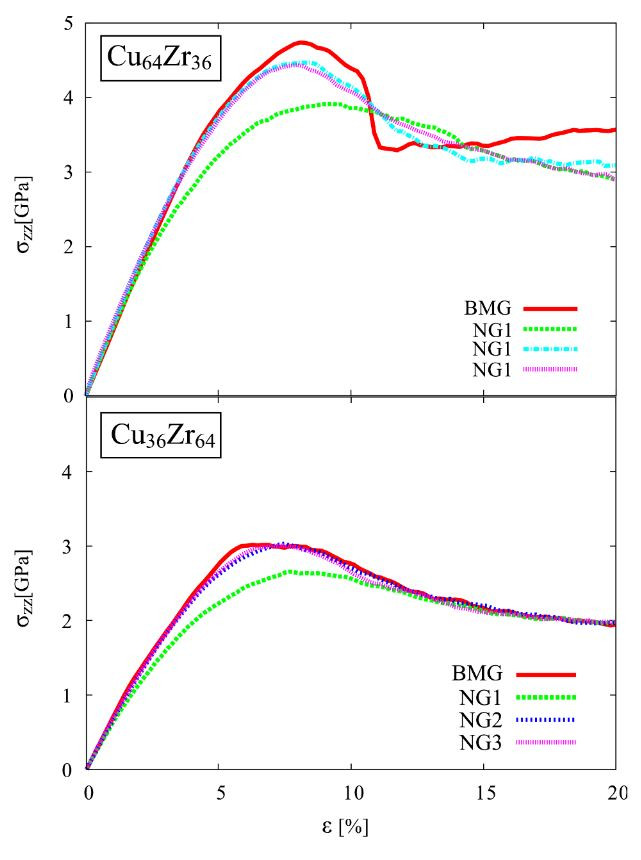Search

# Investigating Structural Properties of Cu-Zr Bulk Metallic Glasses using Molecular DynamicsFigure 1: MD Snapshot (Cu - Blue, Zr - Yellow)

## INTRODUCTION

Molecular Dynamics (MD) is a computational simulation tool that is used to model the dynamic evolution of an interacting system of particles over a period of time. Often, these particles are atoms and molecules that are allowed to interact over a period of time to predict their physical movement. Most commonly, the trajectories of these particles are determined by solving the Newtonian classical equations of motion where the forces between them, and their potential energies are calculated using Interatomic Potentials (IP). This is in contrast to Density Functional Theory (DFT), a similar computational simulation tool, that is based on the principles of Quantum Mechanics. MD is mostly applied to computational chemistry, physics, materials science, and biophysics.

For the purpose of this study, MD is used to investigate the physical structure and properties of a CuZr Bulk Metallic Glass (BMG) system. First, the stress-strain behavior was modeled of 4 CuZr binary compositions: Cu50Zr50, Cu55Zr45, Cu60Zr40, and Cu65Zr35. Following, the structure of the system was investigated using Radial Distribution Function and Voronoi Tessellation Analysis.

## METHOD

Classical molecular dynamics simulations were carried out using LAMMPS molecular dynamic simulator (1). A Finnis-Sinclair Embedded Atom Method (EAM) potential is used to describe Cu-Zr interactions (2). This potential accurately reproduces the structure Cu-Zr amorphous compositions. For the structure generation, a face-centered cubic reference crystal was heated up to 2000K using an isobaric-isothermal (NPT) ensemble. After annealing the structure at 2000K, it was quenched down to room temperature in steps of 25K. At 300K, the structure was equilibrated further for at least 20 ps and was used for further studies. Structural analysis of each composition (Radial Distribution Function and Voronoi analysis) was carried out using OVITO visualization tool (3).

## RESULTSFigure 2: Stress-Strain curve of CuZr compositions simulated using MD.

Stress-Strain Behaviour: Figure 2 shows the simulated Stress/Strain curve of the chosen compositions from the CuZr BMG system. The Stress/Strain curve shows a relationship between the stress applied to a material and the resulting strain (deformation) caused by it. The unit for stress here is 10^9 N/m^2 and the strain is measure in percentage elongation with respect to the original length. The curve can primarily be divided into two regions, linear and non-linear, each of which contains crucial information about the mechanical properties of the material. The slope of the linear portion of the curve can be used to derive the Young’s Modulus (a measure of elasticity) of the material, while the end of a linear trend in the curve denotes the Yield Strength (a measure of limit to which a material can be elastically deformed). On the other hand, the non-linear region can give measurements such as Ultimate Tensile Strength (fracture point of the material). Using these definitions, Young’s Modulus, Yield Strength, and Ultimate Tensile Strength of the chosen alloys were calculated, the values of which are listed in Table 1.Table 1: Mechanical properties of the CuZr compositions simulated using MD.

The stress/strain data derived from MD simulations show increasing Young’s Modulus, Yield Strength, and Ultimate Tensile Strength, with increasing Cu content in the alloy. This is consistent with the Şopu’s MD findings, where the three measured properties increase drastically when Cu is increased from 36% to 64%. Based on the stress/strain curve by Şopu (as shown by the red curve in Figure 3), the Young’s Modulus of Cu64Zr36 and Cu36Zr64 is 89 GPa and 63 GPa respectively, which is consistent with the trends in simulation results within a reasonable margin of error (4). To establish the accuracy of these simulations, simulated Young’s Modulus of Cu50Zr50 (~79 GPa) can be compared to previously reported experimental values, which are in the range of 78 GPa to 87 GPa.Figure 3: Effect of variable Cu content on stress-strain curve of CuZr compositions.

Another thing to note here is the effect of strain rate on the resulting stress-strain curve and therefore on the measured mechanical properties. Several studies observed that the yield strength and ultimate tensile strength increase with increasing strain rate (5). The available strain rates during experimentation range from 10^-4 /s – 10^4 /s while those in MD can be of order 10^8 /s. Since the strain rate is significantly higher during MD simulations, the resulting yield strength and ultimate tensile strength values are expected to be 10% - 20% higher than experimental values.Radial Distribution Function: The Radial Distribution Function (RDF) in a system of particles describes the probability of finding a particle at a distance r away from a given reference particle. The value g(r), as shown in Figure 4, is known as the correlation function. Experimentally, g(r) is obtained through X-Ray Diffraction (XRD), which provides crucial knowledge about the structure of the system. A crystalline system generally has several well-defined peaks which correspond to individual crystalline phases; however, these peaks are absent or wide spread in amorphous solids such as BMGs. The g(r) vs r graph shown here was simulated using MD and provides information about the structure of CuZr BMGs. Firstly, the absence of any sharp peaks confirms a fully amorphous structure of the system, as is expected in BMGs. Secondly, of the first peaks of RDF, the first peak of Cu50Zr50 is the biggest and that of Cu65Zr45 is the smallest, implying that the structural stability of the amorphous structure (as is the case with BMGs) increases with Cu concentration in the sample increasing. This is consistent with the findings of MD studies done previously (6). The first peak of the RDF indicates the average distance at which we find the nearest neighboring atom Cu-Zr(r) i.e., between unlike atoms.Figure 5: Voronoi Polyhedral Fraction

Voronoi Tessellation Analysis: Voronoi Tessellation Analysis (VTA) is a technique used to quantify the local atomic structure or short-range order (SRO) of crystalline and amorphous solids. Polyhedrons are constructed around an atom using its nearest neighbors to understand its coordination characteristics. Regular icosahedrons give an efficient packing compared to distorted icosahedrons. Thus, the lattice strain for the atoms will be lower in structures with a higher fraction of regular icosahedrons. From this analysis, it is observed that the fraction of regular icosahedrons goes up as the Cu content is increased, which suggests an increasing trend in the stability of the alloy system. This is consistent with the observation made using the radial distribution function in the section above (7).

## CONCLUSION

As demonstrated in this whitepaper, MD can serve as a powerful and versatile rule to investigate several properties of alloy systems. While this whitepaper explores the use of MD to investigate structural and mechanical properties, MD can also be used to explore the thermodynamics of a system as well as other kinds of properties. Here, the mechanical properties, such as Young's Modulus and Tensile Strength, were investigated by simulating the Stress-Strain curve for the chosen near-eutectic Cu-Zr binary compositions. It was observed that the strength and elasticity of the material increased with increasing Cu content, consistent with the findings of previous experimental and computational studies. Radial Distribution Function and Voronoi Tessellation analysis also suggested an improvement in the structural stability of the amorphous alloy with increasing Cu content, consistent with previous findings. To conclude, MD offers an accurate and robust means to investigate material compositions without a need for experimental evaluation.

Phaseshift Technologies is a materials development company that develops novel high-performance alloys using Machine Learning and Computational Chemistry. As demonstrated in this study, MD serves as one of several tools Phaseshift uses to develop the chemistry of alloys and guide the experimental efforts. Constantly iterating and developing new and unique computational workflows, Phaseshift aims to reduce the time requirement and cost of materials research and development. The company currently comprises a team of Materials Scientists, Machine Learning Engineers, and Physicists, with a highly specialized set of domain expertise in high-performance alloys.

## REFERENCES

1. LAMMPS Molecular Dynamics Simulator. [Online] [Cited: ] https://lammps.sandia.gov/.

2. Development of suitable interatomic potentials for simulation of liquid and amorphous Cu-Zr alloys. M.I. Mendelev, M.J. Kramer, R.T. Ott, D.J. Sordelet, D. Yagodin, and P. Popel. 11, s.l. : Philosophical Magazine, 2009, Vol. 89, pp. 967-987.

3. Visualization and analysis of atomistic simulation data with OVITO – the Open Visualization Tool Modelling. Stukowski, A. 1, s.l. : Modelling and Simulation in Materials Science and Engineering, 2010, Vol. 18.

4. Influence of grain size and composition, topology, and excess free volume on the deformation behavior of Cu–Zr nanoglasses. Albe, D. Şopu and K. s.l. : Beilstein J. Nanotechnol, 2015, Vol. 6, pp. 537-545.

5. Strain Rate Effect on Tensile Behavior for a High Specific Strength Steel: From Quasi-Static to Intermediate Strain Rates. W. wang, Y. Ma, M. Yang, P. Jiang, F. Yuan, and X. Wu. s.l. : MDPI Open Access Journals, 2017.

6. Mechanical behavior of Cu—Zr bulk metallic glasses (BMGs): A molecular dynamics approach. M. Imra, F. Hussain, M. Rashid, Y. Cai, and S. A. Ahmad. 9, s.l. : Chinese Physics B, 2013, Vol. 22.

7. Origin of the plasticity in bulk amorphous alloys. J. Lee, K. Park, K. Kim, E. Fleury, B. Lee, M. Wakeda, and Y. Shibutani. s.l. : Journal of Material Research, 2007.